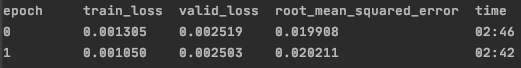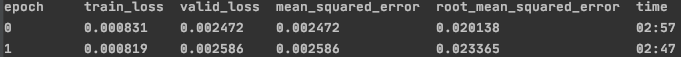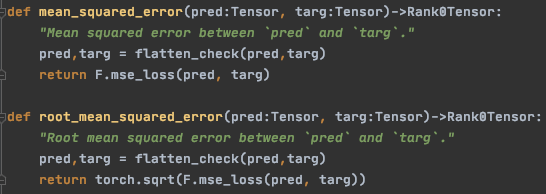# Bug in root_mean_squared_error display? (valid_loss and rmse metric diverge)

For some epochs of training the valid_loss will increase and at the same time the root mean square error metric will decrease and vice versa. It is my understanding that the tabular model uses mean squared error for the valid loss in regression tasks. Hence it does not make sense for them to move in different directions.

I am training a tabular model on a very similar problem to the Rossmann forecasting example.I have looked at the tabular model source code to figure out what loss function its uses and can only find the loss function being assigned to F.nll_loss which wouldn’t make sense for a regression problem as i understand it.
In basic_data.py:

The only reasons I can think for the diverging valid_loss and rmse metric is that they using different segments of data (i thought they both used the valid data set) or it isn’t actually the mse being used to calculate the valid_loss.

Any help on this would be much appreciated I’m struggling to nail the reason myself and it is making it difficult to compare specifications when I have two metrics telling me different things ie I am not sure which one to trust as the final influencer of my specification.

Many thanks

Alex

Possible bug in root_mean_squared_error display?

The valid_loss is definitely the mean_squared_error see image below. However the corresponding root_mean_squared error does not seem to correspond to the mse?Looking at the source code of the metrics you can see the only difference is the use of torch.sqrt. see image below. But applying torch.sqrt to the displayed mse does not yield the rmse and even if this does not balance because it is the average of the mse over the batch it still does not make sense that they diverge on certain epochs?Hi Alexander,

Perhaps this: